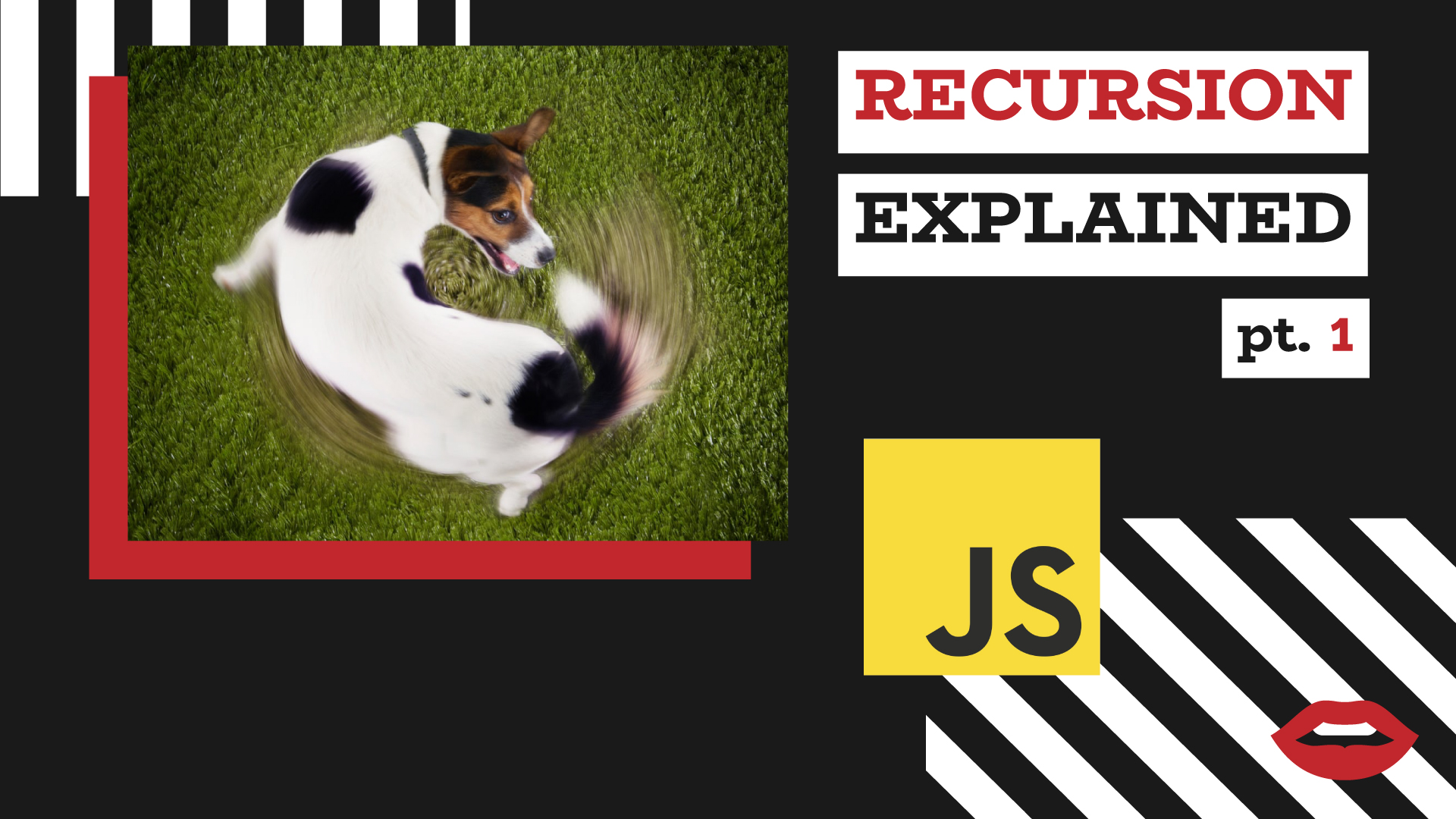# Unlock the power of recursion by understanding the concepts behind itHave you ever struggled, figuring out what recursion means? This post is just for you then! I’ll focus on the principles and ideas behind it rather than default examples (however, I’ll need one to explain) so that you won’t need to memorize any boring theory about it anymore.

Lets jump right in!

This post will be the first part of the two-parts series about the recursion in javascript. Here, I want to share some ideas of how you can think about the recursion.
In the second article, I’ll focus on the practical aspects of it.

## TL;DR

1. There exists two ways of thinking: recursive and iterative.
2. When solving problems using iterative thinking, we do the same task on each iteration.
3. When solving problems using recursive thinking, we use a base case when we know how to solve the problem, and try to get to this point from any other cases by calling the recursive function from within the recursive function. (Sounds complex, but I’m whetting your appetite :P)

## Recursive vs iterative thinking

In general, there can be two ways of thinking: recursive and iterative.
Iterative thinking is one of the first things we learn, starting to learn to code.
It’s somewhere around the `for loops`.
Recursion, on the other hand, is a more complex concept. Let’s start with a definition of a recursive function.

Recursive function is a function calling itself.

Let’s imagine you want to write a function `pow(x, n)` that will raise the first argument to the natural power (or zero) of the second argument.
First of all lets think what we need to do.

If we would do it manually, we would have something like this:

pow(2,0) = 1
pow(2,1) = 2 * 1 = 2
pow(2,2) = 2 * 2 = 4
pow(2,3) = 2 * 2 * 2 = 8
pow(2,4) = 2 * 2 * 2 * 2 = 16…

So it seems like to raise a number to the natural power, we need to perform multiplication the power amount of time.

Let’s apply `iterative thinking` to solve the problem:

``````function pow(x, n) {
let result = 1;

for (let i = 0; i < n; i++) {
result *= x;
}

return result;
}
``````

It works perfectly fine. However, the recursive solution is way more elegant (at the very least people usually say that).

The main idea behind the recursion is that your function should simplify the task and call itself.

There are two different steps:

1. Recursion base - the most trivial case when you should produce a reliable result,
2. Call the function from inside the function.

The most trivial case in our example is when we don’t do anything at all: pow(2,0) = 1.

For this particular case this will work:

``````if (n === 0) {
return 1;
} else {
// Recursion magic will happen here
}
``````

The most trivial case in our example is when we don’t do anything at all: pow(2,0) = 1. To figure that out, let’s try to think again about what we’re trying to achieve.

Let’s consider the case when n === 1:

We know for sure that 2 1 = 2. Let’s rewrite it this way: 2 = 2 * 1 = 2 * 2 0

Here 2 0 is our recursion base.
(Don’t be overwhelmed, hang with me.)

Okay, we got it. Let’s make it a bit more difficult. n === 2

What we know: 2 2 = 4

How we can rewrite it: 4 = 2 * 2 = 2 * 2 1 = 2 * 2 2 - 1

Okay, too much twos… Let’s see what’s going on when n === 3

What we know: 2 3 = 8

How we can rewrite it: 8 = 2 * 4 = 2 * 2 2 = 2 * 2 3 - 1

Here you can notice that we always return to the previous step. And the recursion base is just the first step, the foundation.

## Let me tell you a joke

Do you know about the kettle principle?

The idea is that you should solve your problems based on what you already know.

A mathematician and a physician got an assignment: to boil one liter of water in a kettle. They have a kettle, a faucet, and a stove. For the first time, they solve the problem identically.

#### What they do is:

1. Fill the kettle with water,
2. Turn the stove on,
3. Put the kettle on it,
4. Wait until the water gets boiled,
5. Turn the stove off.

Now they have a kettle with boiled water. After a while, when the temperature decreased significantly, they are asked to boil the water again.

#### What physician does:

1. Turn the stove on,
2. Put the kettle on it,
3. Wait until the water gets boiled,
4. Turn the stove off.

#### What mathematician does:

1. Empty the kettle
2. Fill the kettle with the water,
3. Turn the stove on,
4. Put the kettle on it,
5. Wait until the water gets boiled,
6. Turn the stove off.

Do you feel the difference? The mathematician has used his prior knowledge to solve the problem. I think, the recursion has similar idea. I’m not asking you to drop all your progress so far.
Just think about the recursion base you have, and that you always want to get back to it.
And on each step, you should iteratively call the function from withing the function to use the power and knowledge you already have.

Okay, I’m done with my stupid jokes. In the next article, I’ll cover some recursion examples :) See you then!

If you have something to add, found a mistake, or just want to chat, reach me out on twitter, or write an email :)